# 数据结构与算法之排序shirley

### 排序算法分析

1. 执行效率：从时间复杂度角度衡量。

2. 内存消耗：从空间复杂度角度衡量。原地排序算法，指空间复杂度为O(1)的算法。

3. 稳定性：如果待排序的序列中存在值相等的元素，经过排序之后，相等元素之间原有的先后顺序不变

﻿

﻿

### 冒泡排序（Bubble Sort）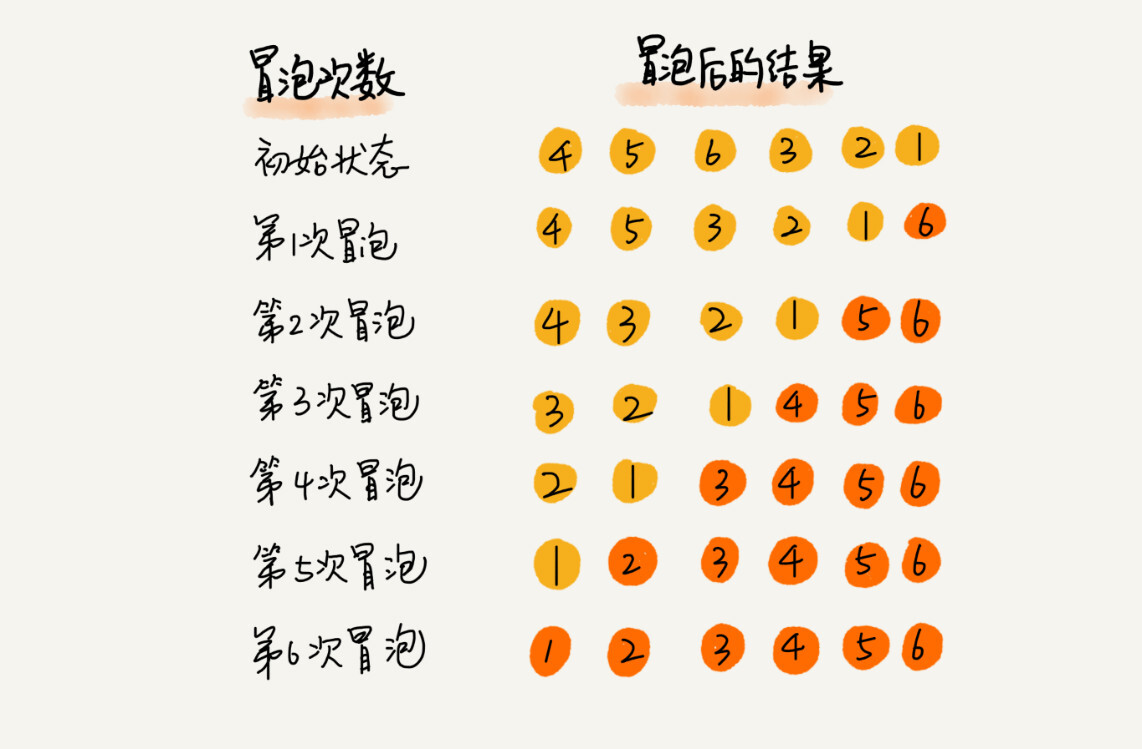﻿

    /**     * 冒泡排序改进:在每一轮排序后记录最后一次元素交换的位置,作为下次比较的边界,     * 对于边界外的元素在下次循环中无需比较.     */     public void bubbleSort(int[] a, int n) {        if (n <= 1) return;        // 最后一次交换的位置        int lastExchange = 0;        // 无序数据的边界,每次只需要比较到这里即可退出        int sortBorder = n - 1;        for (int i = 0; i < n; i++) {            // 提前退出标志位            boolean flag = false;            for (int j = 0; j < sortBorder; j++) {                if (a[j] > a[j + 1]) {                    int tmp = a[j];                    a[j] = a[j + 1];                    a[j + 1] = tmp;                    // 此次冒泡有数据交换                    flag = true;                    // 更新最后一次交换的位置                    lastExchange = j;                }            }            sortBorder = lastExchange;            if (!flag) break;    // 没有数据交换，提前退出        }    }

﻿

### 插入排序（Insertion Sort）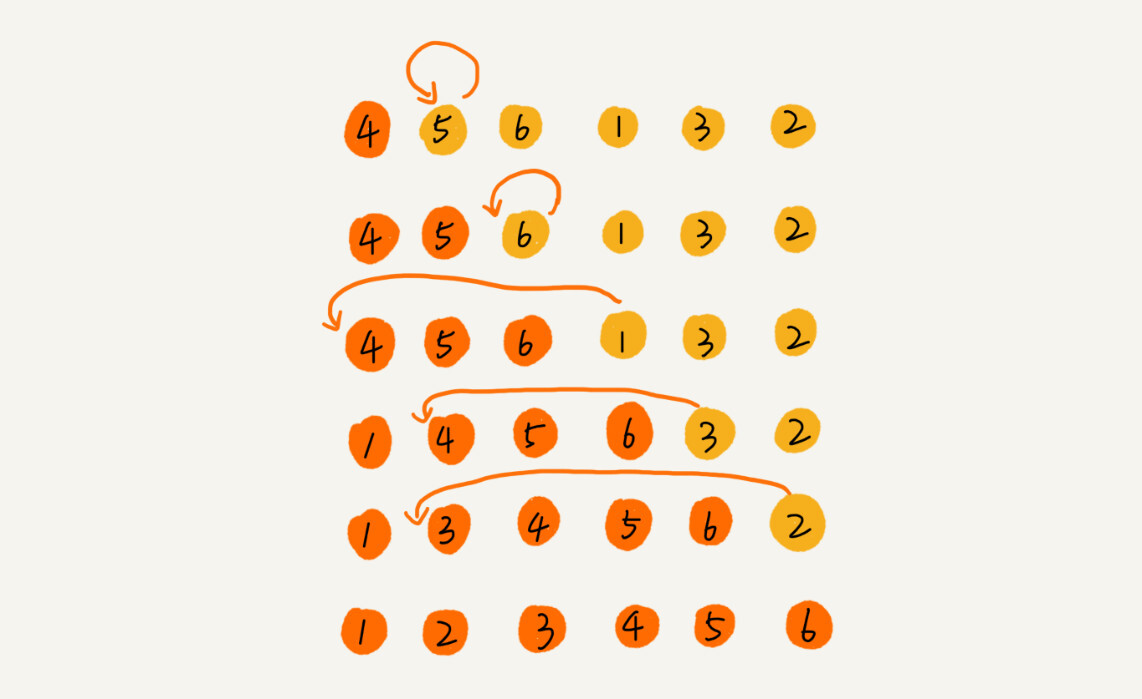// 插入排序，a表示数组，n表示数组大小public void insertionSort(int[] a, int n) {  if (n <= 1) return;  for (int i = 1; i < n; ++i) {    int value = a[i];    int j = i - 1;    // 查找插入的位置    for (; j >= 0; --j) {      if (a[j] > value) {        a[j+1] = a[j];  // 数据移动      } else {        break;      }    }    a[j+1] = value; // 插入数据  }}

﻿

### 选择排序（Selection Sort）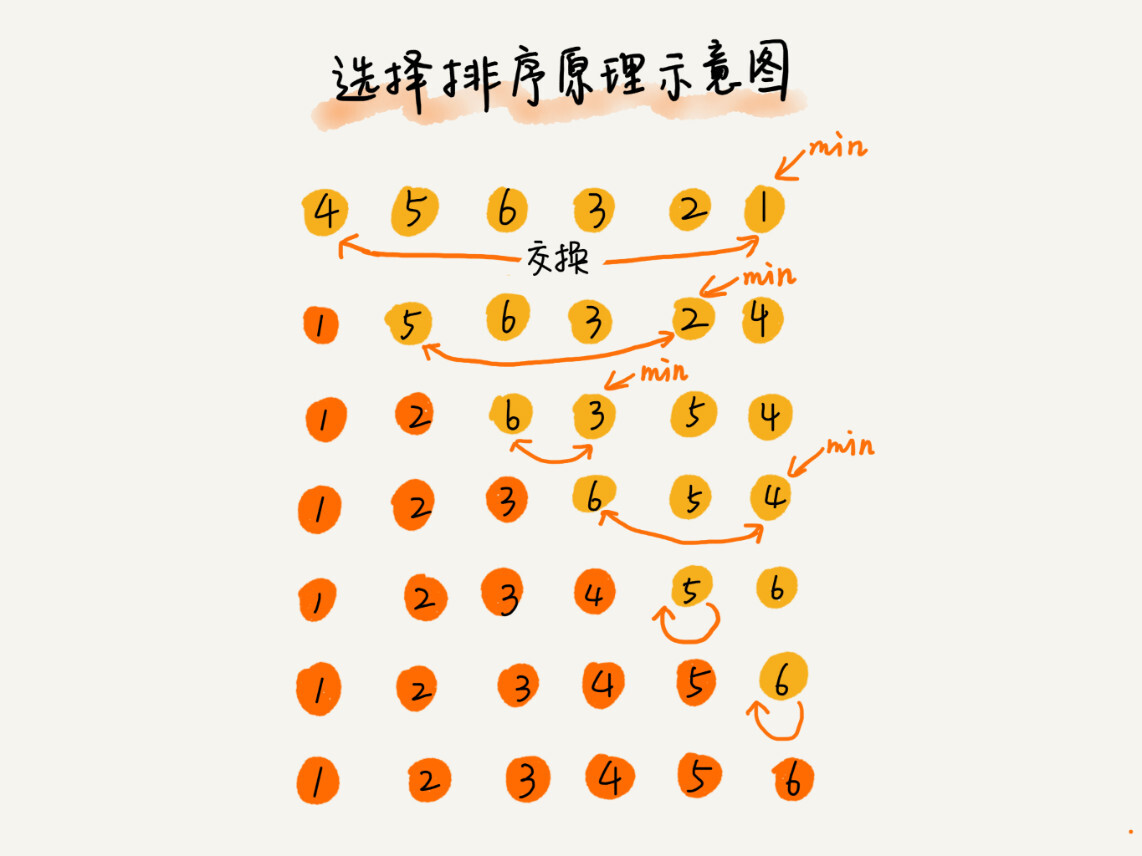// 选择排序，a表示数组，n表示数组大小    public void selectionSort(int[] a, int n) {        if (n <= 1) return;        for (int i = 0; i < n - 1; ++i) {            // 查找最小值            int minIndex = i;            for (int j = i + 1; j < n; ++j) {                if (a[j] < a[minIndex]) {                    minIndex = j;                }            }            // 交换            int tmp = a[i];            a[i] = a[minIndex];            a[minIndex] = tmp;        }    }

﻿

### 归并排序（Merge Sort）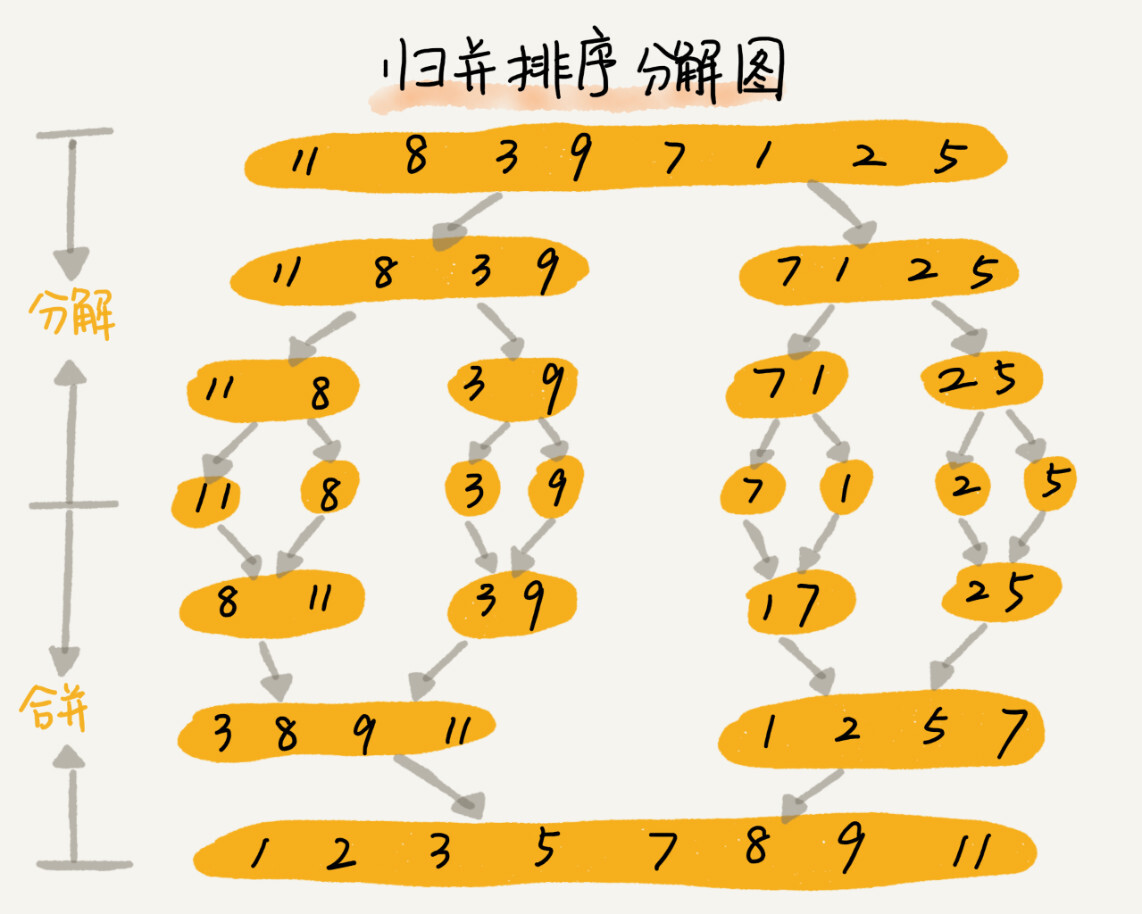﻿

// 归并排序算法, A是数组，n表示数组大小merge_sort(A, n) {  merge_sort_c(A, 0, n-1)}// 递归调用函数merge_sort_c(A, p, r) {  // 递归终止条件  if p >= r  then return  // 取p到r之间的中间位置q  q = (p+r) / 2  // 分治递归  merge_sort_c(A, p, q)  merge_sort_c(A, q+1, r)  // 将A[p...q]和A[q+1...r]合并为A[p...r]  merge(A[p...r], A[p...q], A[q+1...r])}

﻿

merge(A[p...r], A[p...q], A[q+1...r]) {  var i := p，j := q+1，k := 0 // 初始化变量i, j, k  var tmp := new array[0...r-p] // 申请一个大小跟A[p...r]一样的临时数组  while i<=q AND j<=r do {    if A[i] <= A[j] {      tmp[k++] = A[i++] // i++等于i:=i+1    } else {      tmp[k++] = A[j++]    }  }    // 判断哪个子数组中有剩余的数据  var start := i，end := q  if j<=r then start := j, end:=r    // 将剩余的数据拷贝到临时数组tmp  while start <= end do {    tmp[k++] = A[start++]  }    // 将tmp中的数组拷贝回A[p...r]  for i:=0 to r-p do {    A[p+i] = tmp[i]  }}

﻿

### 快速排序（Quick Sort）

﻿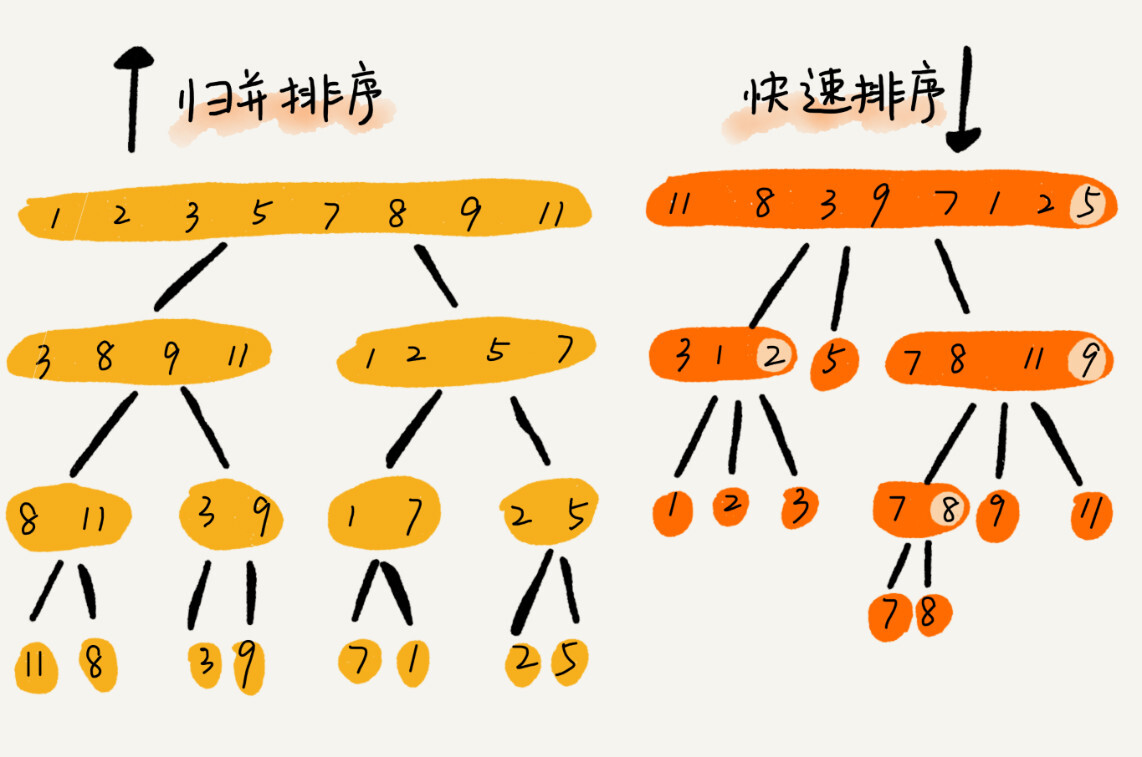﻿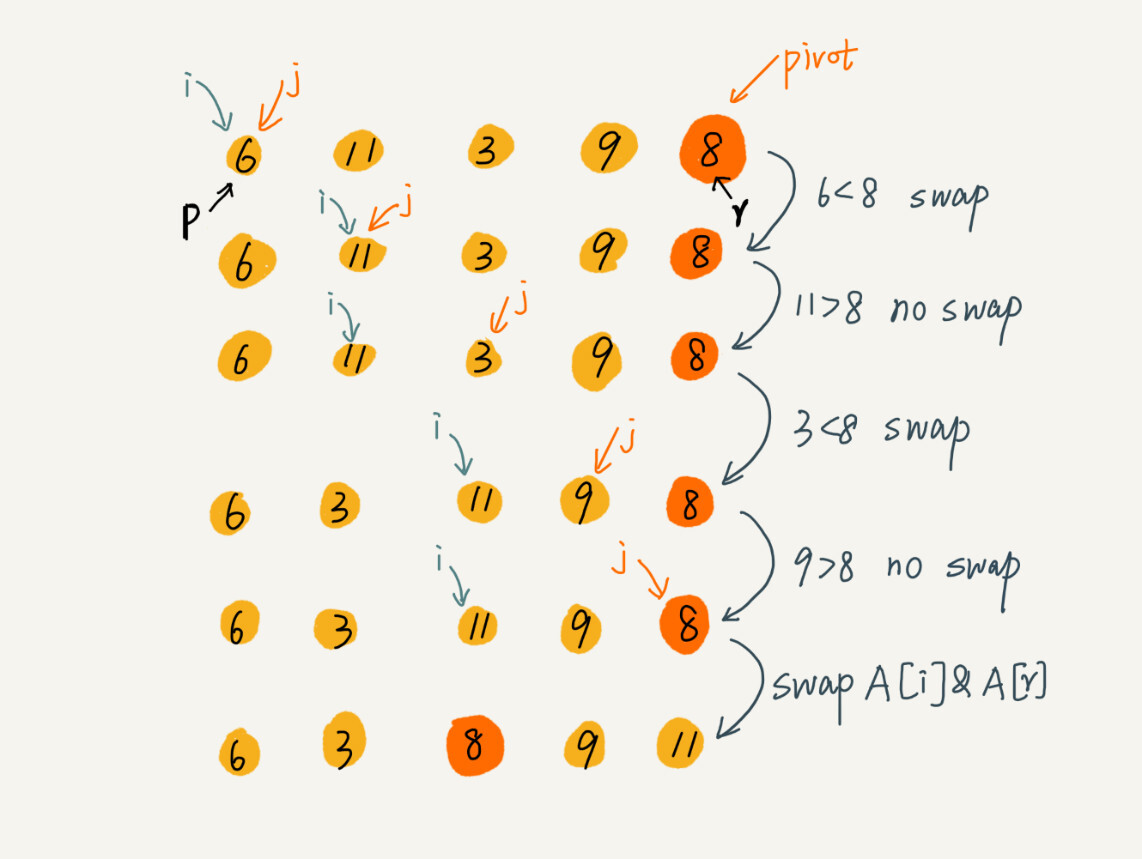﻿

// 快速排序，A是数组，n表示数组的大小quick_sort(A, n) {  quick_sort_c(A, 0, n-1)}// 快速排序递归函数，p,r为下标quick_sort_c(A, p, r) {  if p >= r then return    q = partition(A, p, r) // 获取分区点  quick_sort_c(A, p, q-1)  quick_sort_c(A, q+1, r)}partition(A, p, r) {  pivot := A[r]  i := p  for j := p to r-1 do {    if A[j] < pivot {      swap A[i] with A[j]      i := i+1    }  }  swap A[i] with A[r]  return i

﻿

﻿

### 堆排序（Heap）

#### 堆概念

• 堆是一个完全二叉树；

• 堆中每一个节点的值都必须大于等于（或小于等于）其子树中每个节点的值。

﻿

﻿

﻿

﻿

• 插入一个数据时，把新插入的数据放到数组的最后，然后从下往上堆化

• 删除堆顶数据时，把数组中的最后一个元素放到堆顶，然后从上往下堆化

﻿

#### 堆排序

##### 建堆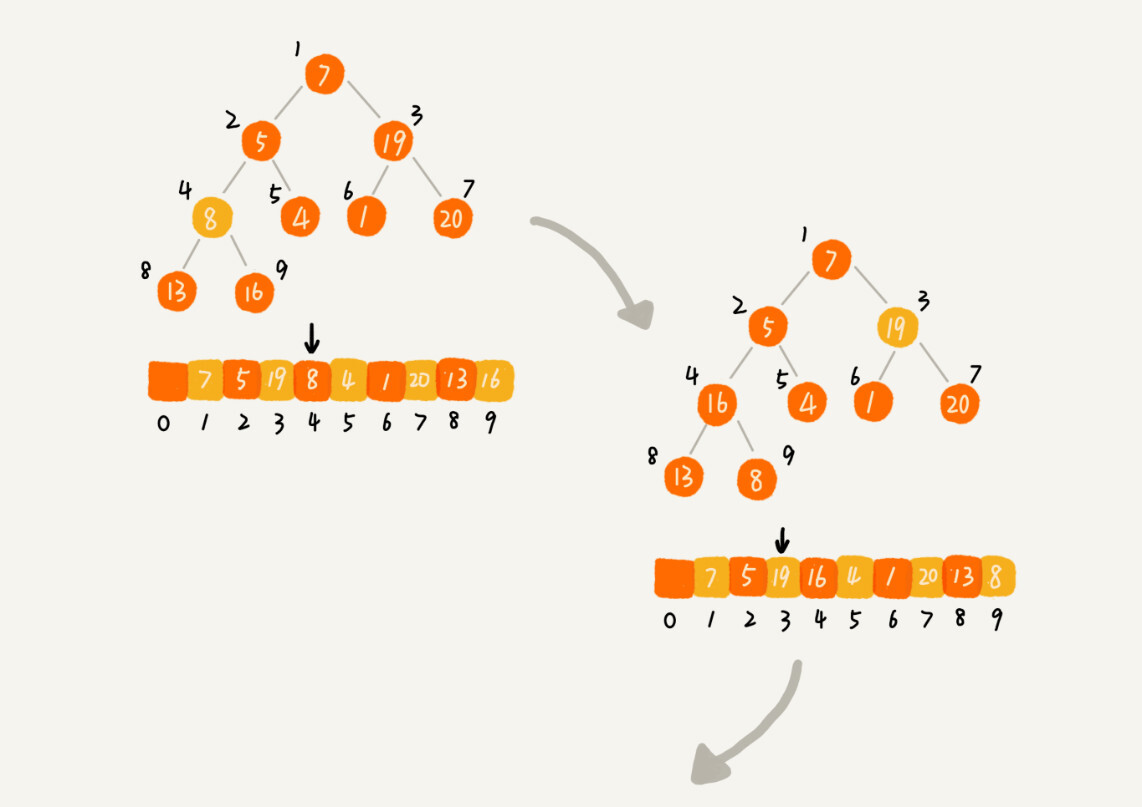private static void buildHeap(int[] a, int n) {  for (int i = n/2; i >= 1; --i) {    heapify(a, n, i);  }}private static void heapify(int[] a, int n, int i) {  while (true) {    int maxPos = i;    if (i*2 <= n && a[i] < a[i*2]) maxPos = i*2;    if (i*2+1 <= n && a[maxPos] < a[i*2+1]) maxPos = i*2+1;    if (maxPos == i) break;    swap(a, i, maxPos);    i = maxPos;  }}
##### 排序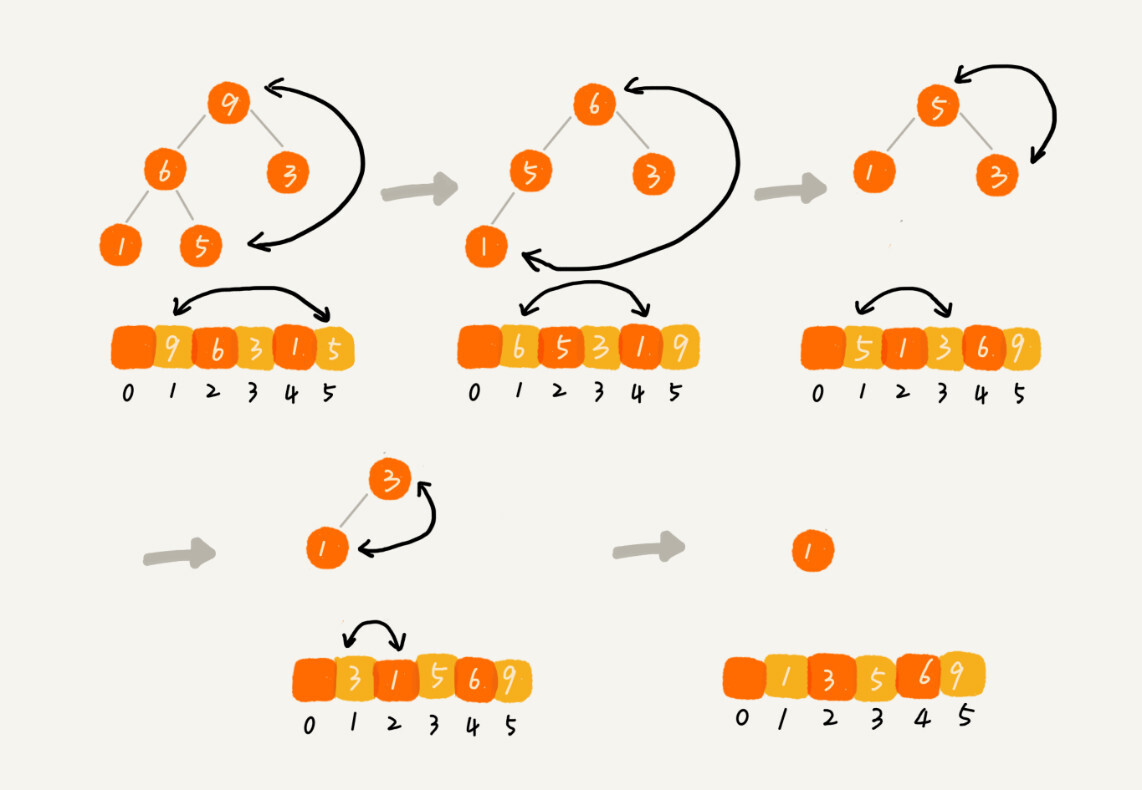﻿

// n表示数据的个数，数组a中的数据从下标1到n的位置。public static void sort(int[] a, int n) {  buildHeap(a, n);  int k = n;  while (k > 1) {    swap(a, 1, k);    --k;    heapify(a, k, 1);  }}

#### 堆的应用

1. 优先级队列：队列是先进先出，不过，在优先级队列中，数据的出队顺序不是先进先出，而是按照优先级来，优先级最高的，最先出队。

1. Top K

2. 求中位数

﻿## 评论